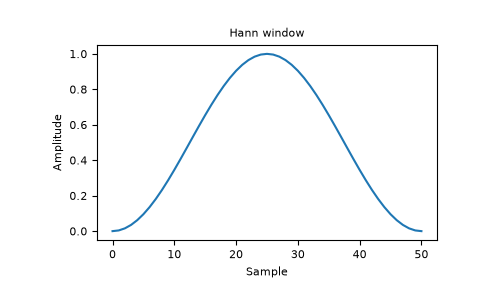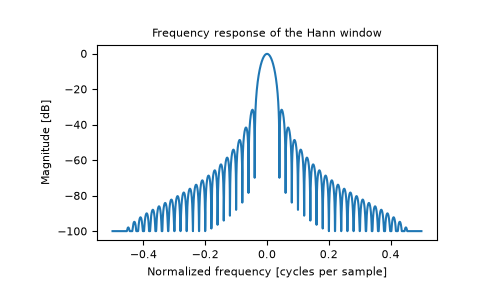numpy.hamming

numpy.kaiser

# numpy.hanning¶

numpy.hanning(M)[source]

Return the Hanning window.

The Hanning window is a taper formed by using a weighted cosine.

Parameters: M : int Number of points in the output window. If zero or less, an empty array is returned. out : ndarray, shape(M,) The window, with the maximum value normalized to one (the value one appears only if M is odd).

Notes

The Hanning window is defined asThe Hanning was named for Julius von Hann, an Austrian meteorologist. It is also known as the Cosine Bell. Some authors prefer that it be called a Hann window, to help avoid confusion with the very similar Hamming window.

Most references to the Hanning window come from the signal processing literature, where it is used as one of many windowing functions for smoothing values. It is also known as an apodization (which means “removing the foot”, i.e. smoothing discontinuities at the beginning and end of the sampled signal) or tapering function.

References

  Blackman, R.B. and Tukey, J.W., (1958) The measurement of power spectra, Dover Publications, New York.
  E.R. Kanasewich, “Time Sequence Analysis in Geophysics”, The University of Alberta Press, 1975, pp. 106-108.
  Wikipedia, “Window function”, https://en.wikipedia.org/wiki/Window_function
  W.H. Press, B.P. Flannery, S.A. Teukolsky, and W.T. Vetterling, “Numerical Recipes”, Cambridge University Press, 1986, page 425.

Examples

>>> np.hanning(12)
array([0.        , 0.07937323, 0.29229249, 0.57115742, 0.82743037,
0.97974649, 0.97974649, 0.82743037, 0.57115742, 0.29229249,
0.07937323, 0.        ])


Plot the window and its frequency response:

>>> import matplotlib.pyplot as plt
>>> from numpy.fft import fft, fftshift
>>> window = np.hanning(51)
>>> plt.plot(window)
[<matplotlib.lines.Line2D object at 0x...>]
>>> plt.title("Hann window")
Text(0.5, 1.0, 'Hann window')
>>> plt.ylabel("Amplitude")
Text(0, 0.5, 'Amplitude')
>>> plt.xlabel("Sample")
Text(0.5, 0, 'Sample')
>>> plt.show()>>> plt.figure()
<Figure size 640x480 with 0 Axes>
>>> A = fft(window, 2048) / 25.5
>>> mag = np.abs(fftshift(A))
>>> freq = np.linspace(-0.5, 0.5, len(A))
>>> with np.errstate(divide='ignore', invalid='ignore'):
...     response = 20 * np.log10(mag)
...
>>> response = np.clip(response, -100, 100)
>>> plt.plot(freq, response)
[<matplotlib.lines.Line2D object at 0x...>]
>>> plt.title("Frequency response of the Hann window")
Text(0.5, 1.0, 'Frequency response of the Hann window')
>>> plt.ylabel("Magnitude [dB]")
Text(0, 0.5, 'Magnitude [dB]')
>>> plt.xlabel("Normalized frequency [cycles per sample]")
Text(0.5, 0, 'Normalized frequency [cycles per sample]')
>>> plt.axis('tight')
...
>>> plt.show()